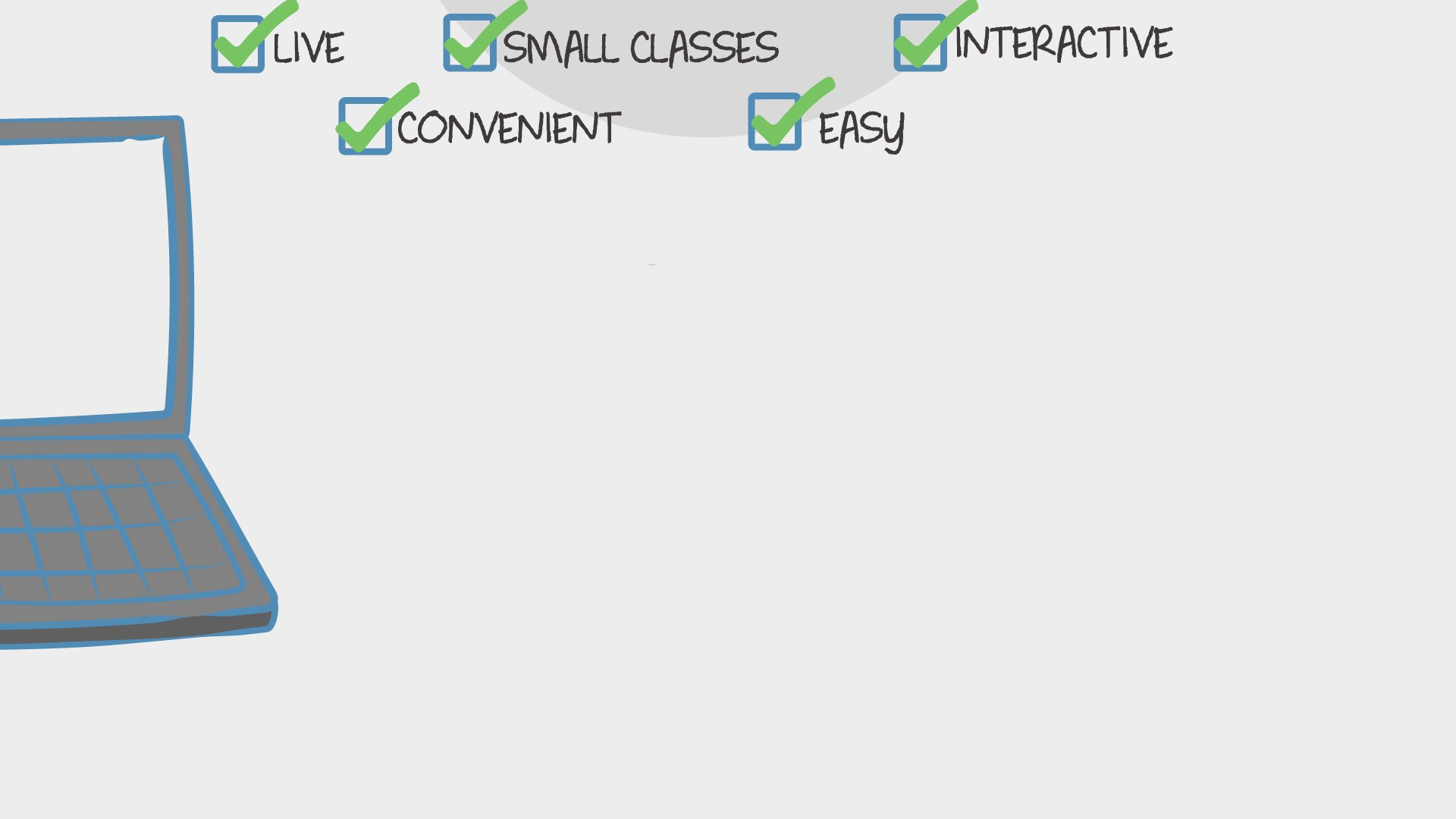Find Classes
Teach

Math

# 3rd Grade Math ( 4th Quarter)

In this 8 week class we will learn 3rd grade, 4th quarter math. I will teach using common core standards.550 total reviews for this teacher
1 review for this class
Completed by 2 learners
There are no upcoming classes.25 minutes
per class
3x per week
over 8 weeks
7-11
year olds
2-6
learners per class
per learner - per class

### How does a “Multi-Day” course work?

Meets multiple times at scheduled times
Live video chats, recorded and monitored for safety and quality
Discussions via classroom forum and private messages with the teacher
Great for engaging projects and interacting with diverse classmates from other states and countries### How Outschool Works### There are no open spots for this class.

You can request another time or scroll down to find more classes like this.

## Description

### Class Experience

```Represent and interpret data.
3.MD.3 Draw a scaled picture graph and a scaled bar graph to represent a data set with several
categories. Solve one- and two-step “how many more” and “how many less” problems using
information presented in scaled bar graphs. For example, draw a bar graph in which each
square in the bar graph might represent 5 pets.
3.MD.4 Generate measurement data by measuring lengths using rulers marked with halves and
fourths of an inch. Show the data by making a line plot, where the horizontal scale is marked
off in appropriate units—whole numbers, halves, or quarters.
3.MD.5 Recognize area as an attribute of plane figures and understand concepts of area
measurement.
a. A square with side length 1 unit, called “a unit square,” is said to have “one square unit”
of area, and can be used to measure area.
b. A plane figure which can be covered without gaps or overlaps by n unit squares is said to
have an area of n square units.
3.MD.6 Measure areas by counting unit squares (square cm, square m, square in, square ft, and
improvised units).
3.MD.7 Relate area to the operations of multiplication and addition.
a. Find the area of a rectangle with whole-number side lengths by tiling it, and show that
the area is the same as would be found by multiplying the side lengths.
b. Multiply side lengths to find areas of rectangles with whole-number side lengths in the
context of solving real world and mathematical problems, and represent whole-number
products as rectangular areas in mathematical reasoning.
c. Use tiling to show in a concrete case that the area of a rectangle with whole-number side
lengths a and b + c is the sum of a × b and a × c. Use area models to represent the
distributive property in mathematical reasoning.
d. Recognize area as additive. Find areas of rectilinear figures by decomposing them into
non-overlapping rectangles and adding the areas of the non-overlapping parts, applying
this technique to solve real world problems.
Solve problems involving the four operations, and identify and explain patterns in
arithmetic.1
3.OA.8 Solve two-step word problems using the four operations. Represent these problems using
equations with a letter standing for the unknown quantity. Assess the reasonableness of
answers using mental computation and estimation strategies including rounding. (This
standard is limited to problems posed with whole numbers and having whole number
answers; students should know how to perform operations in the conventional order when
there are no parentheses to specify a particular order [Order of Operations].)
Represent and interpret data.2
3.MD.4 Generate measurement data by measuring lengths using rulers marked with halves and
fourths of an inch. Show the data by making a line plot, where the horizontal scale is marked
off in appropriate units—whole numbers, halves, or quarters.
Geometric measurement: recognize perimeter as an attribute of plane figures and
distinguish between linear and area measures.
3.MD.8 Solve real world and mathematical problems involving perimeters of polygons, including
finding the perimeter given the side lengths, finding an unknown side length, and exhibiting
rectangles with the same perimeter and different areas or with the same area and different
perimeters.
Reason with shapes and their attributes.3
3.G.1 Understand that shapes in different categories (e.g., rhombuses, rectangles, and others) may
share attributes (e.g., having four sides), and that the shared attributes can define a larger
category (e.g., quadrilaterals). Recognize rhombuses, rectangles, and squares as examples of
quadrilaterals, and draw examples of quadrilaterals that do not belong to any of these
subcategories.```
`Students will need paper and pencil or white board and marker for each class.`
`1 hour 15 minutes per week in class, and maybe some time outside of class.`

## Teacher

`Hi, my name is Madison. I have a Bachelor's of Science Degree in Elementary Education with an emphasis in Social Science and Math. I have a 5 year old son and a two year old daughter. We love to play outside and create art with the things we find... `